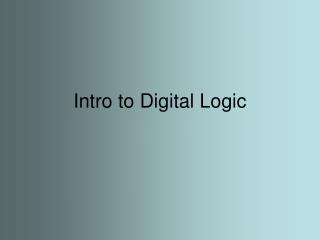DownloadDownload PresentationIntro to Digital Logic

# Intro to Digital Logic

Télécharger la présentation## Intro to Digital Logic

- - - - - - - - - - - - - - - - - - - - - - - - - - - E N D - - - - - - - - - - - - - - - - - - - - - - - - - - -
##### Presentation Transcript

1. Intro to Digital Logic

2. What does “Digital” mean? • A method of storing, processing and transmitting information through the use of distinct electronic or optical pulses that typically represent the binary digits 0 and 1. • This is typically represented by transistors switched “on” or “off” • Data carrying signals carry one of two possible values. • 1/0,on/off,high/low, or pulse present/pulse absent

3. What was the earliest digital communication? • Morse code? • Does it have different states? • 5 different states, but still digital • Semaphore? • What about smoke signals? • One if by air, two if by sea? • All digital, as they are using a code to represent a message

4. Logic! • Why is this unit called digital logic? • What is logic? • Logic in these gates is based on theories from a book called “An investigation in the laws of thought” by George Boole. • This came to be known as Boolean Logic or Boolean Algebra

5. Thoughts • There IS a transition between the low and high, and back to low signals. • In the ideal setting, there would be no transition. • Real world circuits approximate this well enough that we will not take it into consideration

6. Gates • There are seven gates. • The first two are AND and OR gates

7. AND gate • The AND gate logic is such that BOTH outputs must be high to trigger a high output • This is like two doors in a row that both must be open to pass a beam of light through

8. The switch analogy • We can build a manual approximation of the circuit using switches very easily.

9. The OR gate • The OR gate is such that when a high signal is sent on either input, the output will be high. • This is like a double door that either side can open to let the light through.

10. The switch analogy • We can build a manual approximation of the circuit using switches very easily.

11. Positive Logic Vs Negative Logic

12. Positive Logic Vs. Negative Logic • When describing the input states, we can use positive or negative logic • For example, the AND gate: • Positive Logic • When both inputs are high, the output is high • Negative Logic • When either input is low, the output is low

13. Interesting coincidence • On the and gate: • If either input 1 OR input 2 are low, the output is low • OR? How did OR get in there? • This Positive AND gate is also a Negative OR gate! • On an OR gate, both input 1 AND input 2 have to be low in order to have a low output! • AND!? • A positive OR gate is a Negative AND gate!

14. Truth Table (or trooth, if you rather) • A truth table is helpful in determining what type of gate you have, or what you should expect based on your gate • A and B represent the two inputs • C represents output • What type of gate is this?

15. Lab Time

16. Materials • Power Supply (Use +5V DC) • Digital Display Module • Bread Board • Interface Module 2 • Data Switches • Quad AND Gate • Quad OR Gate • Ribbon Cable • Power Supply Leads • Wire

17. How to set it up • Plug the AND gates and Data switches together so they share a common strip in between. • Plug in the Interface Module 2 in above the 2 other modules. • Run a ground wire from the ground side of the AND gate to the ground on the Interface Module. • Plug on end of the ribbon cable into the Interface Module and the other into the DDM on J2 • Turn on power supply and set to +5v DC and hook the leads to the + and – side of the circuit.

18. Build a Single AND Gate Build a truth table for this circuit

19. Build a two AND Gate Circuit Build a truth table for this circuit

20. Build a three AND Gate Circuit Build a truth table for this circuit

21. Build a Single OR Gate Build a truth table for this circuit

22. Build a two OR Gate Circuit Build a truth table for this circuit

23. Build a circuit with one AND and one OR Build a truth table for this circuit